# Adding 3-digit numbers (no regrouping) | 2nd grade | Khan Academy

##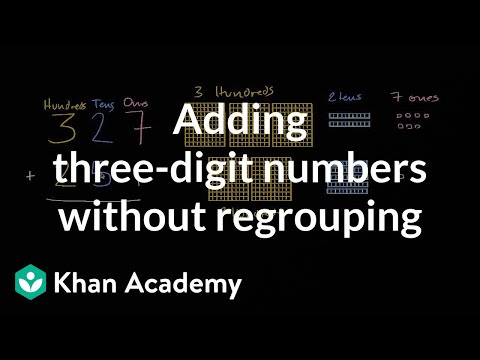By Khan Academy

Sal adds 327 + 251 thinking about place value.# Strategies for adding 2-digit numbers | 2nd grade | Khan Academy

##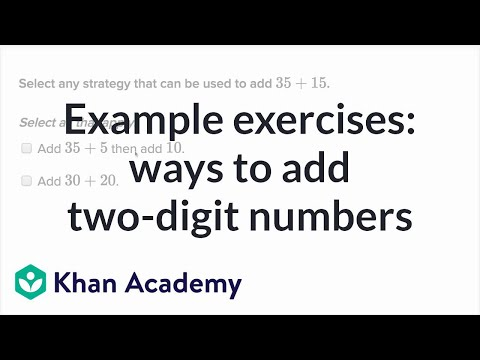By Khan Academy

Sal adds and subtracts numbers like 585 and 368 using a number line. Numbers used in these problems are less than 1000.# Dividing mixed numbers

##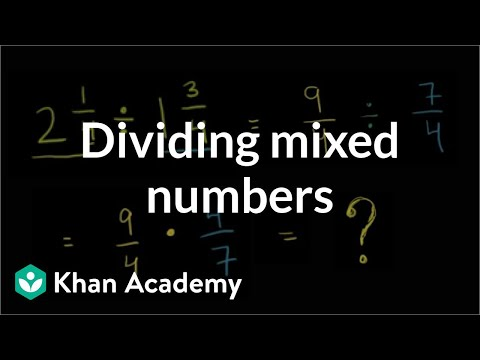By Khan Academy

Dividing Mixed Numbers# Adding two- and three-digit numbers (no regrouping)

##By Khan Academy

Sal shows how to add 327 + 251.# Adding two-digit numbers by making tens

##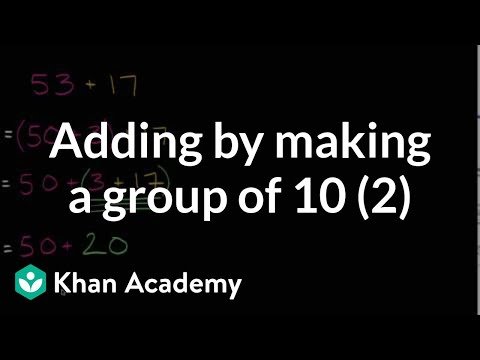By Khan Academy

Learn how to add 53+17 by breaking it apart and making a group of 10.# Adding two-digit numbers by making tens 2

##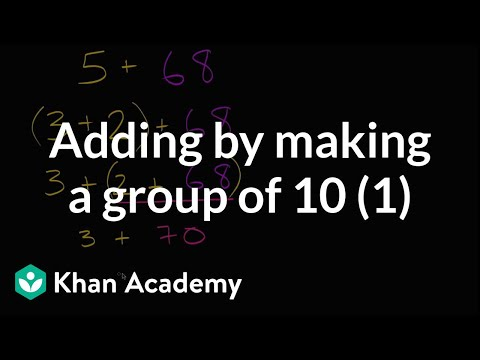By Khan Academy

Learn how to add 5+68 by breaking the 5 into a 2 and a 3.# How to Pass the Math FSA - Area (Addition and Multiplication) (3rd)

##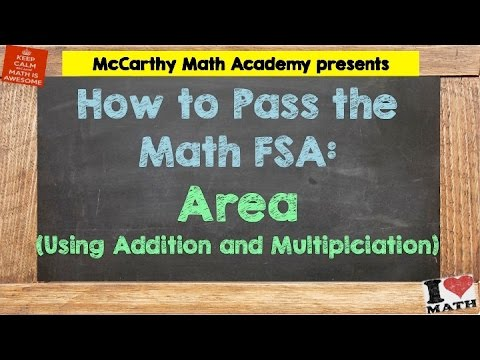By McCarthyAcademy

how to solve 3rd Grade FSA Math-style problems.# Running distance in a week | Multiplication and division

##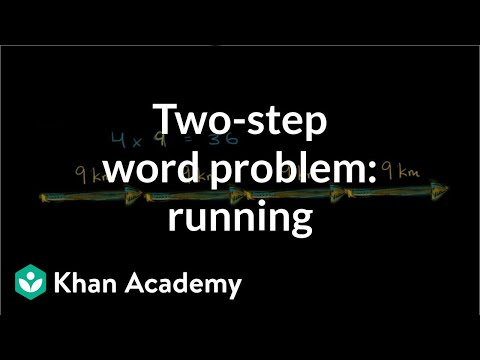By Khan Academy

Solve a two-step word problem by drawing a picture and creating an equation.# Total seats in a theater | Multiplication and division

##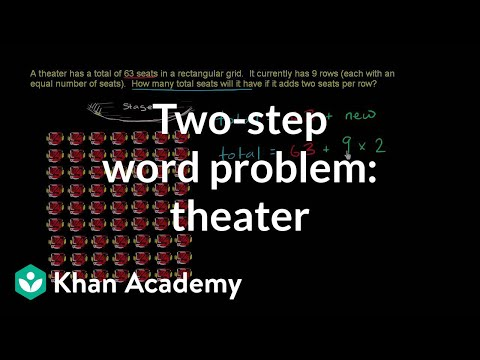By Khan Academy

Solve a two-step word problem by drawing a picture and creating an equation.# Marbles for friends | Multiplication and division

##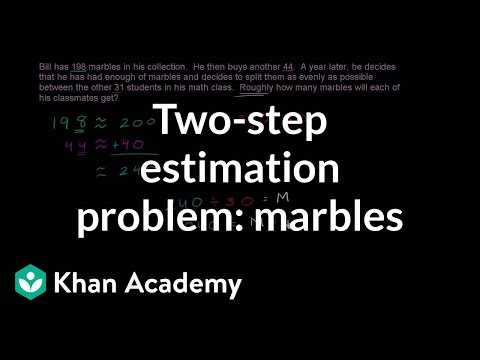By Khan Academy

Solve a two-step estimation word problem.# Grade 2 Math 9.5, Understanding place value (three-digits)

##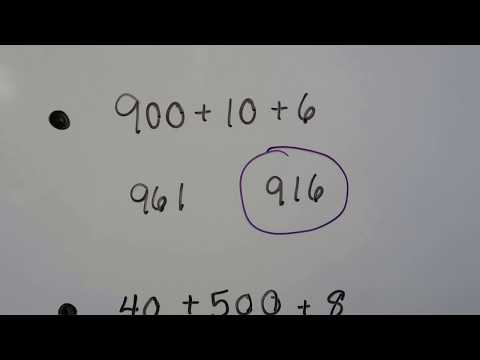By Joann's School

How each digit in a three-digit number has a different value as hundreds, tens, or ones. Expanded form of a three-digit number. Identifying the value of a digit in a three-digit number. Counting by hundreds, then writing the missing number by using a pattern. Writing a number represented by models of hundreds, tens, and ones# Adding three digit numbers with regrouping

##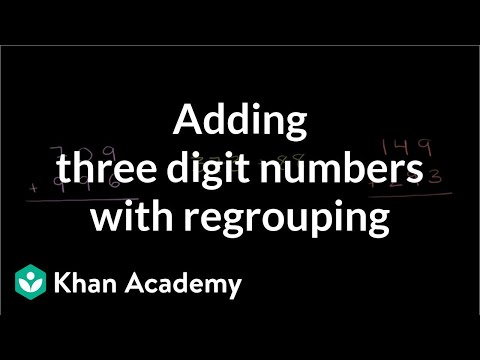By Khan Academy

In the old days 'regrouping' was known as carrying. It's the same idea now as then: moving the tens (or hundreds or thousands) over to the next column.# Division using place value understanding

##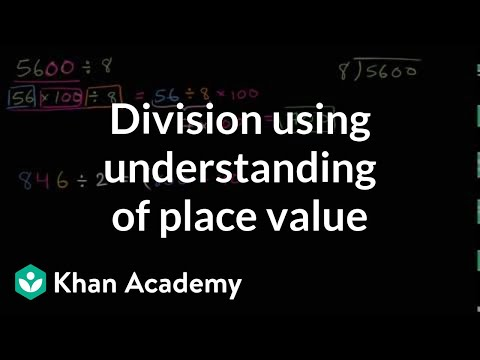By Khan Academy

Make division problems easier by thinking about place value and using the distributive property.# How many truffle eating guests attended a party

##By Khan Academy

Solve a two-step word problem by drawing a picture and creating an equation.# Understanding Numbers and Place Value (4.NBT.2)

##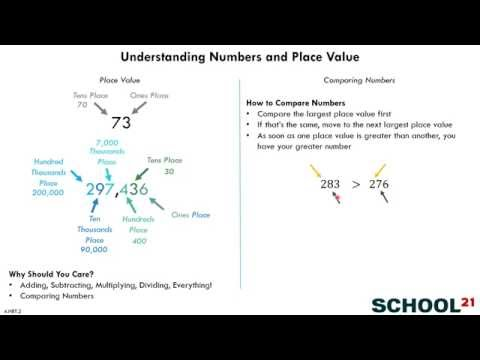By School 21

Read and write multi-digit whole numbers using base-ten numerals, number names, and expanded form# Three-digit place value challenge

##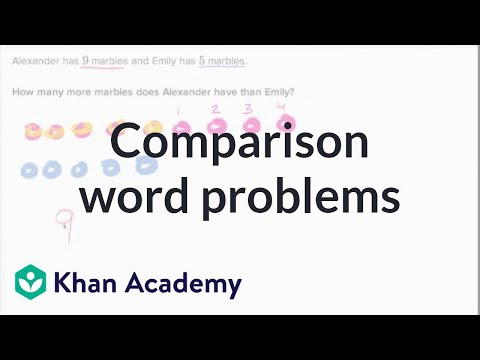By Khan Academy

Word problems with more and fewer,1499# Regrouping numbers intro various place values | Arithmetic properties | Pre-Algebra | Khan Academy

##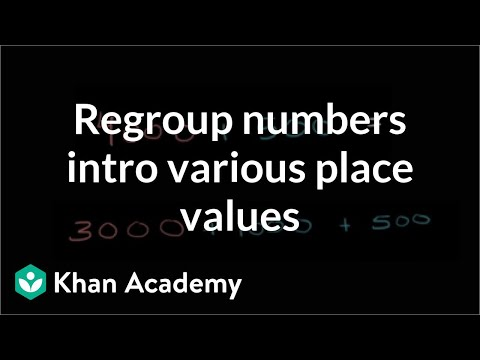By Khan Academy

Thinking about numbers as expressions of different place values is really helpful. In this example we'll look at regrouping a number by different place values.# 4.NBT.1 Place Value of Multi-Digit Whole Numbers Grade 4 Math Lesson

##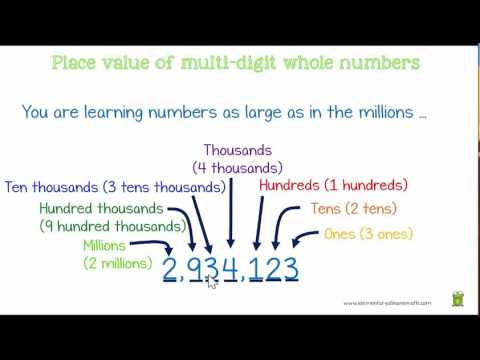By Elemenary All in One Path

Place value of whole number# MathCraft: Place Value Divsion Common Core 4 NBT A1

##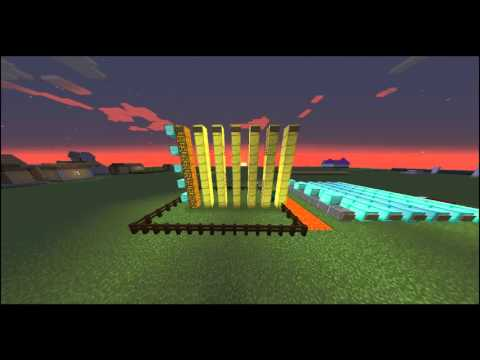By James Pike

Recognize that in a multi-digit whole number, a digit in one place represents ten times what it represents in the place to its right# Multiplying Decimals - On a Place Value Chart (5.NBT.B.7)

##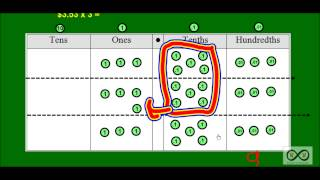By Worksheets and Walkthroughs

This video walkthrough lesson with corresponding worksheets investigates what happens on a place value chart as we multiply a decimal by a single digit number in a story problem format. Place value disks are used to represent values on a place value chart.